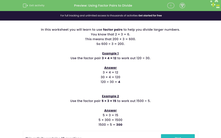# Use Factor Pairs to Solve Division Problems

In this worksheet, students will use factor pairs to help them divide larger numbers.Key stage:  KS 2

Curriculum topic:   Number: Multiplication and Division

Curriculum subtopic:   Recognise/Use Pairs in Mental Calculation

Difficulty level:#### Worksheet Overview

In this activity, we will be using factor pairs to help us divide larger numbers.

To answer the question 600 ÷ 3

We know that 2 × 3 = 6

We also know that 20 x 3 = 60

This means that 200 × 3 = 600

So 600 ÷ 3 = 200

Example 1

Use the factor pair 3 × 4 = 12 to work out 120 ÷ 30

3 × 4 = 12

30 × 4 = 120

120 ÷ 30 = 4

Example 2

Use the factor pair 5 × 3 = 15 to work out 1,500 ÷ 5

5 × 3 = 15

5 x 30 = 150

5 × 300 = 1,500

1,500 ÷ 5 = 300Let's have a go at some questions now and remember that you can look back at this introduction at any point during the questions by clicking on the red help button on the screen.

### What is EdPlace?

We're your National Curriculum aligned online education content provider helping each child succeed in English, maths and science from year 1 to GCSE. With an EdPlace account you’ll be able to track and measure progress, helping each child achieve their best. We build confidence and attainment by personalising each child’s learning at a level that suits them.

Get started